# Divertissement mathématique

One day, driving for some hundreds Km and for many hours, I let my mind going around the vast fields of knowledge and it came back with an interesting demonstration of a well known mathematical formula.

As you know the explicit formulas to solve the equations of any degree becomes immediately complex with the increasing of the equations degree and, more than that, it is possible to provide an explicit formula only for equations of 2°, 3° and 4° degree. This thing, and the difficulty of “deriving” them, have been creating in me some un-comfort since my days in high school.

The formula of 2° degree is already very complex and I’m sure 99% of you does not even remember it.

here it is: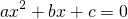has the following solutions:I remember that the demonstration was based on adding and subtracting quantities that magically allowed to simplify and aggregate the equation in a way that can be solved. I never liked this procedure since I found it very artificial. So I had this little thing in the back of my mind for years and years.

So I started thinking about a simple equation of which everybody knows the solution:where the solutions are clearlyand I was thinking a way to use this knowledge and build something out of it to get to the generalized solution.

And indeed I found a nice way to do so and do not get crazy with the strange demonstration that is given at school.

The idea is to insert the expression of a I degree general equation in that: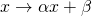so that we can try solving the following: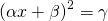we obtain:and then..and finally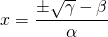Interesting… The resolution formula is amazingly similar to the general one, there should be something good in it!

Let’s take again the equation I used and let’s expand it:or..That is extremely similar to:we need to impose: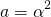If we are able to calculate α, β, γ as functions of a, b, c, maybe we can derive the general formula without strange complications…

Well, we obtain indeed:and then:one more step:I was amazed to see that it was possible to revert the equations!

I’m now able to calculate the general formula:turns to be:that can be written as:that becomes: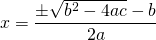This methodology allows to derive the formula to solve a II degree equation with a  two step approach: the first is to calculate the square root ofand the second is to solve a I degree equation.

This is very interesting: maybe it is possible to do the same and derive the solving formula for a IV degree equation starting from a II degree equation by solving: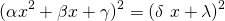This IV degree equation can be solved easily and on the same time can be equated easily to a generic IV equation such asif the resulting system that links a,b,c,d,e with α,β,γ,δ,λ can be solved, the resulting formulas should be derived. The resulting formula should look like as a composition of a double II solving formula with one “nested” in the other as the equation in α,β,γ,δ,λ is a II degree equation nested in another II degree equation. I know, mathematics will cry that I’m crazy in saying so….

Looking at the solving formulas it looks like that it is the case:Source Wikipedia

the equations looks pretty nice. I have not derived them from the methodology I propose. If anybody wants to use mathematica to do so and is willing to share the calculation, I would appreciate very much. If anybody wants to support me, I would appreciate. For the moment I faced only a situation in which the equations to solve are 4th grade… so circular argument…

This suggest to me that the procedure wont’ work further, as clearly stated by a well known theorem that says that there are not explicit solving formulas for equation of an higher degree that IV.

The following two tabs change content below.

#### Andrea Terzaghi

Geneva (CH)
Ingegnere, pellegrino del mondo e della conoscenza, curioso di tutto

#### Ultimi post di Andrea Terzaghi (vedi tutti)

Questo articolo è stato pubblicato in Excel, Matematica e contrassegnato come da Andrea Terzaghi . Aggiungi il permalink ai segnalibri.## Informazioni su Andrea Terzaghi

Ingegnere, pellegrino del mondo e della conoscenza, curioso di tutto